Next: Electric Field of a Up: Gauss' Law Previous: Gauss' Law

## Electric Field of a Spherical Conducting Shell

Suppose that a thin, spherical, conducting shell carries a negative charge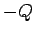. We expect the excess electrons to mutually repel one another, and, thereby, become uniformly distributed over the surface of the shell. The electric field-lines produced outside such a charge distribution point towards the surface of the conductor, and end on the excess electrons. Moreover, the field-lines are normal to the surface of the conductor. This must be the case, otherwise the electric field would have a component parallel to the conducting surface. Since the excess electrons are free to move through the conductor, any parallel component of the field would cause a redistribution of the charges on the shell. This process will only cease when the parallel component has been reduced to zero over the whole surface of the shell. It follows that:
The electric field immediately above the surface of a conductor is directed normal to that surface.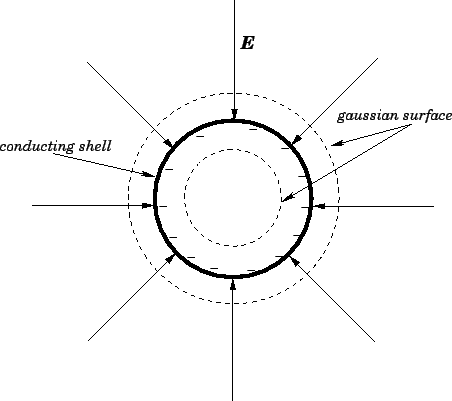Let us consider an imaginary surface, usually referred to as a gaussian surface, which is a sphere of radiuslying just above the surface of the conductor. Since the electric field-lines are everywhere normal to this surface, Gauss' law tells us that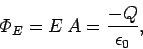(68)

where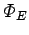is the electric flux through the gaussian surface,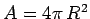the area of this surface, andthe electric field-strength just above the surface of the conductor. Note that, by symmetry,is uniform over the surface of the conductor. It follows that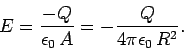(69)

But, this is the same result as would be obtained from Coulomb's law for a point charge of magnitudelocated at the centre of the conducting shell. Now, a simple extension of the above argument leads to the conclusion that Eq. (69) holds everywhere outside the shell (withrepresenting the radial distance from the center of the shell). Hence, we conclude the electric field outside a charged, spherical, conducting shell is the same as that generated when all the charge is concentrated at the centre of the shell.

Let us repeat the above calculation using a spherical gaussian surface which lies just inside the conducting shell. Now, the gaussian surface encloses no charge, since all of the charge lies on the shell, so it follows from Gauss' law, and symmetry, that the electric field inside the shell is zero. In fact, the electric field inside any closed hollow conductor is zero (assuming that the region enclosed by the conductor contains no charges).Next: Electric Field of a Up: Gauss' Law Previous: Gauss' Law
Richard Fitzpatrick 2007-07-14